Triangular array : Array « Collections Data Structure « Java

Triangular array

```
import java.text.Format;

public class TriangularArray {
public static void main(String[] args) {
final int MAX_HIGH = 10;

// allocate triangular array
int[][] odds = new int[MAX_HIGH + 1][];
for (int i = 0; i <= MAX_HIGH; i++)
odds[i] = new int[i + 1];

// fill triangular array
for (int i = 0; i < odds.length; i++)
for (int j = 0; j < odds[i].length; j++)
odds[i][j] = i+j;

// print triangular array
for (int i = 0; i < odds.length; i++) {
for (int j = 0; j < odds[i].length; j++){
System.out.print(odds[i][j] + " ");
}
System.out.println();

}
}
}

```

Related examples in the same category

 1 Initialize a static array 2 Initialization and re-assignment of arrays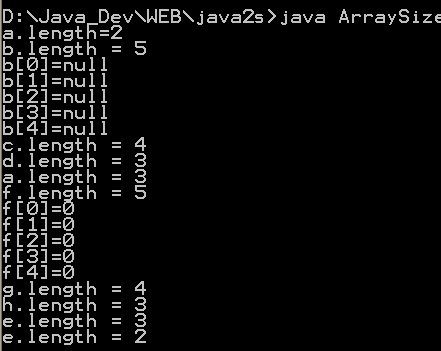3 Doubling the size of an array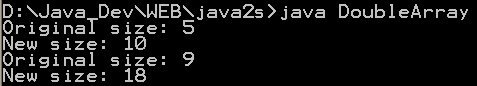4 Timing array loop performance 5 Array 2D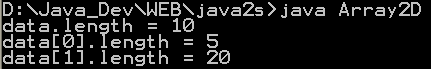6 Can you change the .length of an array 7 Show Two-Dimensional Array of Objects 8 ArrayListDemo done over using an ArrayList 9 Array Hunt game 10 Multi Dimension Array 11 Clone Array 12 Associates keys with values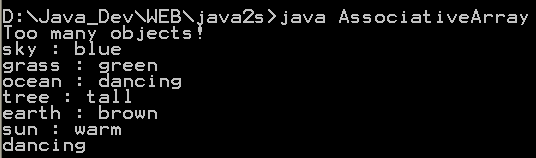13 Arrays of primitives 14 Creating arrays with new 15 Array initialization 16 Creating an array of nonprimitive objects 17 Create multidimension arrays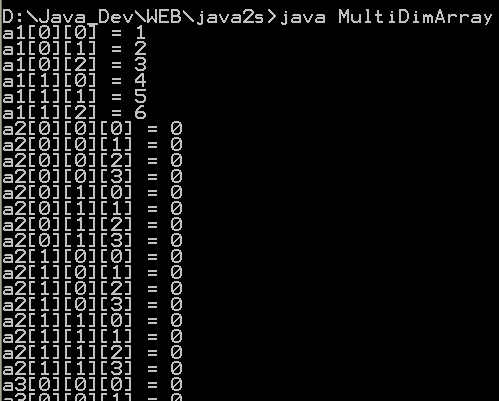18 Initializing Array Values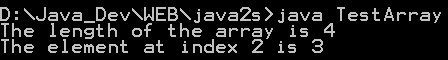19 Creating a Two-Dimensional Array 20 Initializing a Two Dimensional Array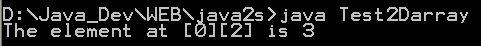21 Using the length Variable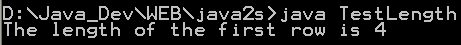22 Grow array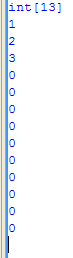23 Define array for class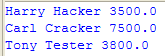24 String array and output to console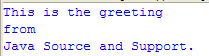25 Multiply two matrices 26 Array Of Arrays Demo 2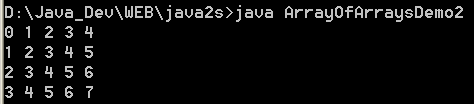27 Array Copy Demo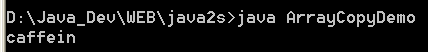28 Copying Elements from One Array to Another 29 Java program to demonstrate multidimensional arrays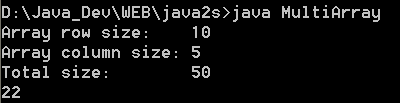30 Extend the size of an array 31 Copy an array 32 Initialize multidimensional array 33 Get array upperbound 34 To get the number of dimensions 35 Resize an array, System.arraycopy() 36 Dump array content: Convert the array to a List and then convert to String 37 java.utils.Arrays provides ways to dump the content of an array. 38 Dump multi-dimensional arrays 39 Use the new shorthand notation to iterate through an array 40 Create a repeated sequence of character 41 Reverse array elements order 42 Convert array of primitives into array of objects 43 Array Initializers 44 Reinitializes a byte array 45 Reinitializes an int array 46 Sum all elements in the array 47 Sums an array of numbers log(x1)...log(xn) 48 A class to iterate over all permutations of an array. 49 Palidrome Array 50 Set of utilities used to manipulate arrays. 51 ArrayUtils provides static methods for manipulating arrays when using a tool such as java.util.ArrayList is inconvenient. 52 Array Util 53 clone two dimensional array